# Contact scheme

contact network, contact circuit, switching circuit

A representation of a class of special control systems, one of the mathematical models of actual structures built from relay contacts. A contact network is a modelling class of control systems, and the same problems are considered for it as for other classes of control systems; it is particularly useful in the study of the "structural" properties of control systems.

A contact networkis obtained by attaching to each edge of some graph with selected vertices one of the letters of a finite alphabet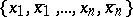. The selected vertices are called the terminals or nodes of the network. An edge with a letter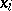(or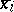) attached to it is called a make (respectively, break) contact. A sequence of contacts between two terminalsandofcorresponding to a simple chain (or path) (see Graph) in the graph ofis called a chain betweenand; the conjunction of the corresponding letters is called the conductivity of the given chain. The conductivity between two terminalsandof the scheme is a Boolean function, equal to the disjunction of the conductivities of all chains between these terminals (when the set of chains betweenandis empty,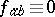; whencoincides with,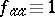). Associated with each contact network is a conductivity matrix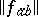, the elements of which are the conductivities between pairs of terminals. Here,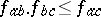. Conversely, given a matrix of Boolean functionssuch that,and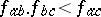for any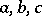, there exists a contact network with given terminals for which the conductivities are the given. In particular, there exists for anya two-terminal network the conductivity between the terminals of which is. In this case one says that the network realizes the function. For example, the network of Fig. arealizes the linear function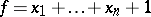(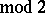).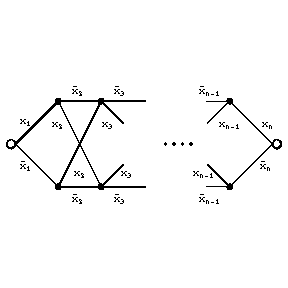Figure: c025460a

Every Boolean function is realized by some contact network. Sometimes the set of all terminals in a contact network is divided into two subsets, the inputs and outputs. A contact network withinputs andoutputs is called a contact-terminal network. A contact network the conductivity between any pairs of outputs (or inputs) of which is zero, is called separating with respect to its outputs (or inputs). An example of a separating (with respect to outputs)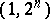-terminal network is given by a contact tree (Fig. b).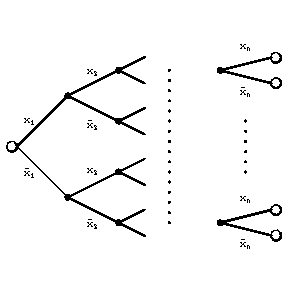Figure: c025460b

A contact network is called planar if its graph with a source edge (that is, the edge joining the terminals to which no letter of the alphabet is attached) added is planar (see Graph, planar). A planar contact networkis said to be dual to a planar contact networkif the graph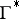of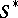(with source edge) is dual to the corresponding graphof, where the source edge ofcorresponds to that of, while the remaining corresponding edges are ascribed the same letters (Fig. c).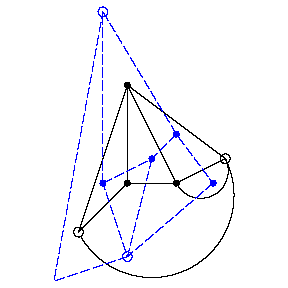Figure: c025460c

The networksandhave the same number of contacts and realize dual functions (the duality principle). If the contacts of a networkare replaced by their opposites, one obtains a network for the negation of the function realized by. In general, it is not possible to pass from non-planar contact networks to networks with the same number of contacts realizing dual functions. A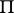-network (parallel-series network) can be defined inductively: A contact network consisting of a single contact joining terminals is a-network; a contact network constructed from two-networks joined in parallel or in series is a-network. There exists contact networks that are not-networks, for example the network of Fig. d.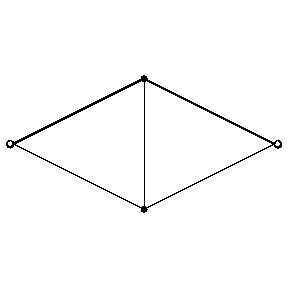Figure: c025460d

The dual of a-network is a-network. There is a correspondence between-networks and formulas in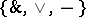. Under this connection every-network realizes the same function as the formula corresponding to it and contains as many contacts as there are letters in the formula. For example, corresponding to the network of Fig. e is the formula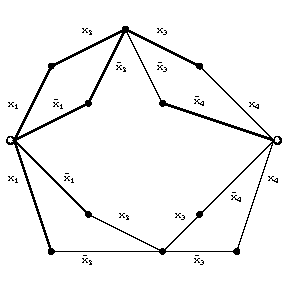Figure: c025460e

The complexity of a contact network is the number of its contacts. The minimum number of contacts that suffice for the realization of an arbitrary Boolean function depending onvariables by a contact network is asymptotically equal to; the minimum number of contacts sufficient for the realization by a-network is asymptotically equal to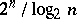(see Synthesis problems).

Two contact networks are said to be equivalent (under a given one-to-one correspondence between their terminals) if the conductivities between any pair of corresponding terminals of these networks are the same. On replacing some subnetwork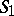of a contact networkby an equivalent one, one obtains a network equivalent to. (In this replacement it is necessary to consider as terminals ofall terminals ofoccurring inand all the vertices ofthat are incident to contacts ofthat are not in.) If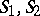are equivalent networks, then the rule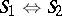of equivalent transformation of contact networks enables one to replace, in any network, a subnetwork obtained from(or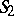) by a renaming of the letters, by the contact network obtained from(or) by the same renaming.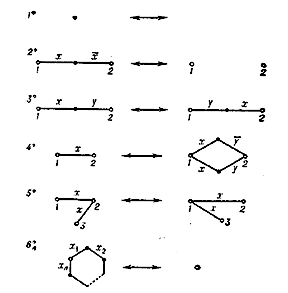Figure: c025460f

There exists for eacha finite complete system of rules (Fig. f) enabling one to transfer between any two equivalent contact networks with number of variables not exceeding. There does not, however, exist for the class of all contact networks (without restriction on the number of variables) a finite complete system of rules (if on applying the rules only renaming of letters is permitted).

How to Cite This Entry:
Contact scheme. N.A. Karpova (originator), Encyclopedia of Mathematics. URL: http://encyclopediaofmath.org/index.php?title=Contact_scheme&oldid=17371
This text originally appeared in Encyclopedia of Mathematics - ISBN 1402006098